# Neural Network Training¶

In this chapter, we'll talk about neural network training, plotting the training curve, and tuning hyper-parameters. Let's start with the neural network training code that we wrote previously:

In :
import torch
import torch.nn as nn
import torch.nn.functional as F
import torch.optim as optim
from torchvision import datasets, transforms
import matplotlib.pyplot as plt # for plotting

torch.manual_seed(1) # set the random seed

class Pigeon(nn.Module):
def __init__(self):
super(Pigeon, self).__init__()
self.layer1 = nn.Linear(28 * 28, 30)
self.layer2 = nn.Linear(30, 1)
def forward(self, img):
flattened = img.view(-1, 28 * 28)
activation1 = self.layer1(flattened)
activation1 = F.relu(activation1)
activation2 = self.layer2(activation1)
return activation2

# load the data
mnist_train = list(mnist_train)[:2000]
img_to_tensor = transforms.ToTensor()

# create a new model, initialize random parameters
pigeon = Pigeon()

# loss and optimizer
criterion = nn.BCEWithLogitsLoss()
optimizer = optim.SGD(pigeon.parameters(), lr=0.005, momentum=0.9)

# training
for (image, label) in mnist_train[:1000]:
# actual ground truth: is the digit less than 3?
actual = (label < 3).reshape([1,1]).type(torch.FloatTensor)
# prediction
out = pigeon(img_to_tensor(image))
# update the parameters based on the loss
loss = criterion(out, actual) # compute the loss
loss.backward()               # compute updates for each parameter
optimizer.step()              # make the updates for each parameter
optimizer.zero_grad()         # a clean up step for PyTorch


## Batching¶

Let's break down the training code again a little more. At each iteration of the main training loop, we:

• we use our network to make the predictions for one image
• we compute the loss for that one image
• we take a "step" to optimize the loss of that one image

In a biological setting, it makes sense to ask real pigeons to reason about one image at a time. However, with an artificial neural network, we may want to use more than one image at one time. That way, we can compute the average loss across a mini-batch of multiple images, and take a step to optimize the average loss. The average loss across multiple training inputs is going to be less "noisy" than the loss for a single input, and is less likely to provide "bad information" because of a "bad" input.

So, we are going to do the following at each iteration:

• we use our network to make the predictions for $n$ images
• we compute the average loss for those $n$ images
• we take a "step" to optimize the average loss of those $n$ images

The number $n$ is called the batch size.

In one extreme, we can set $n = 1$, as we have done above. However, having such a small batch size means that we might be optimizing a very different loss function in each iteration. The "steps" that we make might cause our parameters to change in different directions. Training might therefore take longer because of the noisiness.

In the other extreme, we can set $n$ to be the size of our training set. We would be computing the average loss for our entire training set at every iteration. When we have a small training set, this strategy might be feasible. When we have a large training set, computing the predictions and loss for every training input becomes expensive. Besides, the average loss might not change very much as we keep increasing our batch size.

The actual batch size that we choose depends on many things. We want our batch size to be large enough to not be too "noisy", but not so large as to make each iteration too expensive to run.

People often choose batch sizes of the form $n=2^k$ so that it is easy to half or double the batch size. We'll choose a batch size of 32 and train the network again.

First, we'll use some PyTorch helpers to make it easy to sample 32 images at once:

In :
mnist_train = datasets.MNIST('data', train=True,
transform=img_to_tensor) # apply img_to_tensor to every image


Recall that items in mnist_train looks like this:

In :
img, label = list(mnist_train)
print(img.shape)
print(label)
print(label.shape)

torch.Size([1, 28, 28])
tensor(5)
torch.Size([])


Each image is a tensor of dimension 1 x 28 x 28. Tensors are n-dimensional analogs of matrices. You can think of these as n-dimensional arrays.

Let's take a look at what train_loader does for us:

In :
train_loader_iter = iter(train_loader)
imgs, labels = next(train_loader_iter)
print(imgs.shape)
print(labels)
print(labels.shape)

torch.Size([32, 1, 28, 28])
tensor([5, 0, 4, 1, 9, 2, 1, 3, 1, 4, 3, 5, 3, 6, 1, 7, 2, 8, 6, 9, 4, 0, 9, 1,
1, 2, 4, 3, 2, 7, 3, 8])
torch.Size()


The variable imgs now has 32 images instead of just one.

By design, neural networks are highly parallel, and heavily use matrix operations. (Remember how to compute activations of a neuron based on the previous layer's neuron? The math looked like this: $f(\sum_i w_i x_i)$. The input to the non-linearity $f$ is really just the dot product of two vectors!)

Our new training code actually looks almost identical to our old training code, again because PyTorch does a very good job parallelizing operations.

In :
for n, (imgs, labels) in enumerate(train_loader):
# stop after 10 iterations
if n >= 10:
break
# actual ground truth: is the digit less than 3?
actual = (labels < 3).reshape([32, 1]).type(torch.FloatTensor)
# prediction
out = pigeon(imgs)
# update the parameters based on the loss
loss = criterion(out, actual) # compute the total loss
loss.backward()               # compute updates for each parameter
optimizer.step()              # make the updates for each parameter
optimizer.zero_grad()         # a clean up step for PyTorch


In the code above, we are optimizing the total loss across all examples, as opposed to the average loss. They are the same thing up to multiplication by a constant (the batch size).

## Iterations and Epochs¶

We've been using term iteration as in an iteration of the for loop. However, this term has meaning in neural network training aside from the structure of the code. An iteration in neural network training is one parameter update step. That is, in each iteration, each parameter is updated once.

In our earlier training code at the top of this section, we trained our neural network for 1000 iterations, and a batch size of 1. In our more recent training code, we trained for 10 iterations. We used a batch size of 32, so the actual number of training images we used is 320.

The way train_loader works is that it randomly groups the training data into mini-batches with the appropriate batch size. Each data point belongs to only one mini-batch. When there are no more mini-batches left, the loop terminates.

In general, we may wish to train the network for longer. We may wish to use each training data point more than once. In other words, we may wish to train a neural network for more than one epoch. An epoch is a measure of the number of times all training data is used once to update the parameters. So far, we haven't even trained on a full epoch of the MNIST data.

Both epochs and iterations are units of measurement for the amount of neural network training. If you know the size of your training set and the batch size, you can easily convert between the two.

We want our neural networks to train quickly. We want the highest accuracy or lowest error with the fewest number of iterations or epochs, so that we can save time and electricity. We saw that our choice of batch size can affect how quickly our neural network can achieve a certain accuracy.

## Learning Rate¶

The optimizer settings can also affect the speed of neural network training. In particular, all optimizers have a setting called the learning rate, which controls the size of each parameter update step we take.

In :
optimizer = optim.SGD(pigeon.parameters(), # the parameters that this optimizer optimizes
lr=0.005,            # the learning rate
momentum=0.9)        # other optimizer settings


If we double the learning rate (to lr=0.01), then we will take a step twice the size in each iteration -- or each time we call optimizer.step().

A learning rate too small would mean that the network parameters don't change very much in each iteration. As a result, the network could take a long time to train.

A learning rate too large can mean that we change our parameters a lot, and likely possibly in different directions in different iterations. We might even overshoot when moving the parameters in the appropriate direction, going past an optimal point.

An appropriate learning rate depends on the batch size, the problem, the particular optimizer used (optim.SGD vs a different optimizer), and the stage of training. A large batch size will afford us a larger learning rate, and a smaller batch size requires a smaller learning rate. Practitioners also often reduce the learning rate as training progresses, and as we approach a good set of parameter values.

## Tracking Training using a Training Curve¶

How do we know when to stop training? How do we know what learning rate and what batch sizes are appropriate? Those are very important and practical questions to answer when training a neural network. We answer those questions by plotting a training curve.

A training curve is a chart that shows:

• The iterations or epochs on the x-axis
• The loss or accuracy on the y-axis.

The idea is to track how the loss or accuracy changes as training progresses.

Let's plot a training curve for training a new Pigeon network on the first 1024 training images. We'll use a batch size of 1.

In :
# only use the first 1024 images for training
mnist_data = datasets.MNIST('data', train=True, transform=img_to_tensor)
mnist_data = list(mnist_data)
mnist_train = mnist_data[:1024]

# create a new network with random weights, and optimizer for the network
pigeon = Pigeon()
optimizer = optim.SGD(pigeon.parameters(), lr=0.005, momentum=0.9)

iters  = [] # save the iteration counts here for plotting
losses = [] # save the avg loss here for plotting

# training
for n, (imgs, labels) in enumerate(train_loader):
actual = (labels < 3).reshape([1, 1]).type(torch.FloatTensor)
out = pigeon(imgs)
loss = criterion(out, actual) # compute the loss
loss.backward()               # compute updates for each parameter
optimizer.step()              # make the updates for each parameter
optimizer.zero_grad()         # a clean up step for PyTorch

# save the current training information
iters.append(n)
losses.append(float(loss))

# plotting
plt.plot(iters, losses)
plt.title("Training Curve (batch_size=1, lr=0.005)")
plt.xlabel("Iterations")
plt.ylabel("Loss")
plt.show()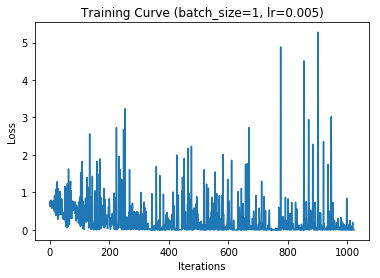The first thing that you might notice is that the loss is very noisy. Some people choose to plot a running average of the loss to remove some of the noise, but I will ask you to just squint for now.

The loss tends to improve very quickly near the beginning of training. To be able to better see the loss improvement towards the middle and end of the epoch, we can omit the first few iterations from our training curve:

Let's see how the training curve changes as we change the batch size and the learning rate. We will still plot one epoch of training with 1024 images, so that the comparison with the earlier plots is fair.

Since we'll be varying the batch size and learning rate, we'll write a function that plots the training curve.

In :
def plot_training_curve(batch_size=1, lr=0.005):
"""
Plots the training curve on one epoch of training of the
Pigeon network trained using the first 1024 images in
the MNIST dataset.
"""

pigeon = Pigeon()
optimizer = optim.SGD(pigeon.parameters(), lr=lr, momentum=0.9)

iters  = []
losses = []

# training
for n, (imgs, labels) in enumerate(train_loader):
actual = (labels < 3).reshape([batch_size, 1]) \
.type(torch.FloatTensor)
out = pigeon(imgs)
loss = criterion(out, actual) # compute the total loss
loss.backward()               # compute updates for each parameter
optimizer.step()              # make the updates for each parameter
optimizer.zero_grad()         # a clean up step for PyTorch

# save the current training information
iters.append(n)
losses.append(float(loss)/batch_size) # compute *average* loss

# plotting
plt.plot(iters, losses)
plt.title("Training Curve (batch_size={}, lr={})".format(batch_size, lr))
plt.xlabel("Iterations")
plt.ylabel("Loss")
plt.show()


### Increasing the Batch Size¶

First, let's try a batch size of 32 and the same learning rate as before

In :
plot_training_curve(batch_size=32, lr=0.005)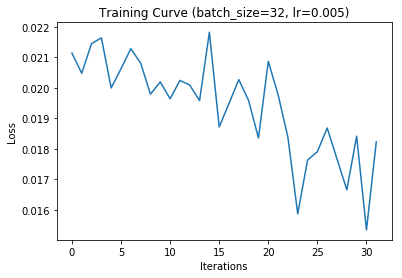The loss curve is a lot less noisy than before, and you can see that the network is still improving (the loss is still on a decline). Despite the general downward trend, the training loss can increase from time to time. Recall that in each iteration, we are computing the loss on a different mini-batch of training data.

### Increasing the Learning Rate¶

Since we increased the batch size, we might be able to get away with a higher learning rate. Let's try.

In :
plot_training_curve(batch_size=32, lr=0.01)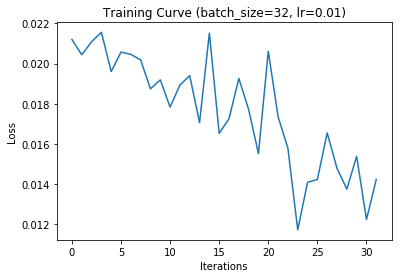The neural network trains much more quickly, and achieves a lower minimum loss.

### Decreasing the Learning Rate¶

For comparison, here's what happens when we decrease the learning rate.

In :
plot_training_curve(batch_size=32, lr=0.001)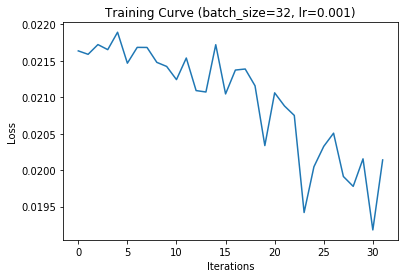The network trains a little slowly, but is less noisy.

## Decreasing Learning Rate with Batch Size 1¶

If we keep the learning rate low, but have our batch size of 1, the noise is also reduced slightly.

In :
plot_training_curve(batch_size=1, lr=0.001)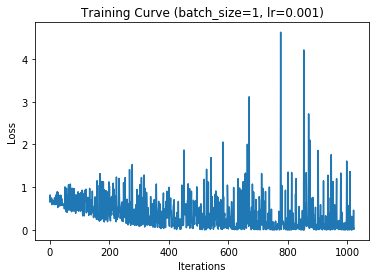## Plotting Error or Accuracy¶

Instead of plotting the loss on the y-axis, we could also choose to plot the training accuracy or training error. The downside is that we would have to compute the training accuracy, which is extra work that we don't normally do during training.

We also have to make a choice: do we compute the accuracy across the minibatch, the entire training set, or some subset of the training set? To be fair, we could also plot the loss for the entire training set instead of for the minibatch as well -- but accuracy and error rates tend to be more "discrete" than loss values, requiring a larger sample to be meaningful.

We could also choose to compute the training accuracy/error every few iterations, as opposed to after every iteration. We would still be able to see the general trend, but without as much cost.

For our purposes, let's first write a general helper function that computes the accuracy of a model across some dataset.

In :
# get a PyTorch tensor of the entire training set, for computing accuracy
all_train_imgs, all_train_labels = next(iter(tmp_loader))
all_train_actual = (all_train_labels < 3).reshape([1024, 1]).type(torch.FloatTensor)

def get_accuracy(model, input=all_train_imgs, actual=all_train_actual):
"""
Return the accuracy of the model on the input data and actual ground truth.
"""
prob = torch.sigmoid(model(input))
pred = (prob > 0.5).type(torch.FloatTensor)
correct = (pred == actual).type(torch.FloatTensor)
return float(torch.mean(correct))


Now, let's revise the plot_training_curve function to plot two curves: one that plots the loss function, and another that plots the accuracy across the entire training set at every iteration.

In :
def plot_training_curve_with_acc(batch_size=1, lr=0.005):
"""
Plots the training curve on one epoch of training
of the Pigeon network trained using the first 1024 images in
the MNIST dataset.
"""

pigeon = Pigeon()
optimizer = optim.SGD(pigeon.parameters(), lr=lr, momentum=0.9)

iters  = []
losses = []
train_acc = []

# training
for n, (imgs, labels) in enumerate(train_loader):
actual = (labels < 3).reshape([batch_size, 1]).type(torch.FloatTensor)
out = pigeon(imgs)
loss = criterion(out, actual) # compute the total loss
loss.backward()               # compute updates for each parameter
optimizer.step()              # make the updates for each parameter
optimizer.zero_grad()         # a clean up step for PyTorch

# save the current training information
iters.append(n)
losses.append(float(loss)/batch_size) # compute *average* loss
train_acc.append(get_accuracy(pigeon)) # compute training accuracy

# plotting
plt.plot(iters, losses)
plt.title("Training Curve (batch_size={}, lr={})".format(batch_size, lr))
plt.xlabel("Iterations")
plt.ylabel("Loss")
plt.show()
plt.plot(iters, train_acc)
plt.title("Training Curve (batch_size={}, lr={})".format(batch_size, lr))
plt.xlabel("Iterations")
plt.ylabel("Training Accuracy")
plt.show()


Here's our best model so far:

In :
plot_training_curve_with_acc(batch_size=32, lr=0.01)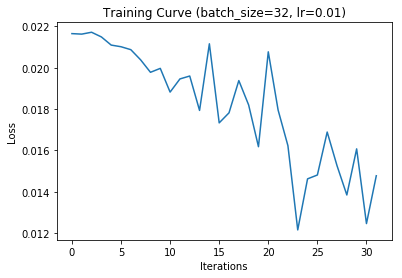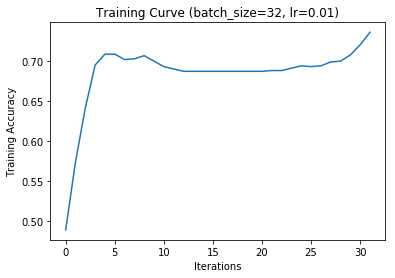The training accuracy curve tells an interesting story. First, the accuracy improves fairly quickly. Then, the accuracy flattens as the loss improves. (This is possible because the loss looks at the continuous probabilities that the network produces, rather than the discrete predictions.) Finally, towards the end of the epoch, the training accuracy improves again.

## Validation Accuracy¶

Towards the end of last week, we discussed how the training accuracy (and, by extension, the training loss) is not a realistic estimate of how well the network will perform on new data. We run into this exact problem with our training curve. At each iteration of training, we know how the network will perform on the data it is trained on, but not on new data. It is difficult to tell whether the network learned to solve the problem at hand, or it simply memorized the training data.

We proposed to set aside a test set at the end of training to determine how our model is doing. We could do something similar here: we use a set of data not used for training to track how well our training is going.

However, we don't want to use our test set for this purpose. The reason is that we may make some decisions about our neural network architecture, training, and settings, using the training curve. We don't want to "contaminate" our test set by using it to make any decision -- otherwise the test set would no longer help us estimate how well our network will perform on unseen data.

We will therefore use a separate dataset called the validation set, separate from both the training set and the test set, to see how well our model is doing in each iteration. The validation set will help us make decisions about the appropriate batch size, learning rate, and the values of other relevant settings. The split between training, validation, and test set is usually 60% training, 20% validation, 20% test. Other splits like 70/15/15, 80/10/10, 50/25/25 are also reasonable, depending on how much data is available.

We can plot the validation accuracy during training, like this:

In :
# get a PyTorch tensor of the entire validation , for computing accuracy
mnist_val = mnist_data[1024:2048] # our choice of validation set
all_val_imgs, all_val_labels = next(iter(tmp_loader))
all_val_actual = (all_val_labels < 3).reshape([1024, 1]).type(torch.FloatTensor)

def plot_training_curve_with_val(model, batch_size=1, lr=0.005, num_epochs=1):
"""
Plot the training curve on num_epochs of training
of the model trained using the first 1024 images in
the MNIST dataset.

Return the trained model.
"""
optimizer = optim.SGD(model.parameters(), lr=lr, momentum=0.9)

iters  = []
losses = []
train_acc = []
val_acc = []

# training
n = 0 # the number of iterations
for epoch in range(num_epochs):
for imgs, labels in iter(train_loader):
actual = (labels < 3).reshape([batch_size, 1]).type(torch.FloatTensor)
out = model(imgs)
loss = criterion(out, actual) # compute the total loss
loss.backward()               # compute updates for each parameter
optimizer.step()              # make the updates for each parameter
optimizer.zero_grad()         # a clean up step for PyTorch

# save the current training information
iters.append(n)
losses.append(float(loss)/batch_size)  # compute *average* loss
train_acc.append(get_accuracy(model)) # compute training accuracy
val_acc.append(get_accuracy(model,    # compute validation accuracy
all_val_imgs,
all_val_actual))
# increment the iteration number
n += 1

# plotting
plt.title("Training Curve (batch_size={}, lr={})".format(batch_size, lr))
plt.plot(iters, losses, label="Train")
plt.xlabel("Iterations")
plt.ylabel("Loss")
plt.show()

plt.title("Training Curve (batch_size={}, lr={})".format(batch_size, lr))
plt.plot(iters, train_acc, label="Train")
plt.plot(iters, val_acc, label="Validation")
plt.xlabel("Iterations")
plt.ylabel("Training Accuracy")
plt.legend(loc='best')
plt.show()

print("Final Training Accuracy: {}".format(train_acc[-1]))
print("Final Validation Accuracy: {}".format(val_acc[-1]))

return model


Let's plot the training curve for batch_size=32, lr=0.005, for 15 epochs of training. Recall that since our batch size is 32, and our training set has size 1024, each epoch corresponds to 1024 / 32 = 32 iterations of training.

In :
pigeon = plot_training_curve_with_val(Pigeon(),
batch_size=32,
lr=0.005,
num_epochs=15)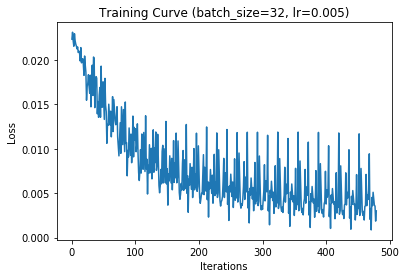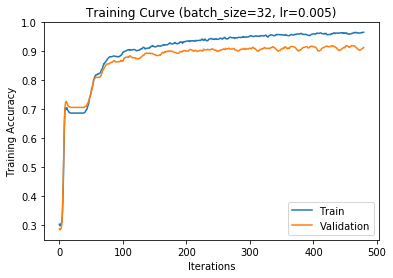Final Training Accuracy: 0.96484375
Final Validation Accuracy: 0.912109375


## Hyper-parameters¶

There are many other neural network settings that affect how well our network will perform, including:

• The size of the network:
• number of layers
• number of neurons in each layer
• Choice of activation function
• Other optimization settings

These settings are called hyper-parameters. They are distinct from parameters in that they are often discrete, and cannot be optimized using an optimizer.

There are many, many hyper-parameters. In theory, we have an infinite choice of neural network models to choose from. In practice, we begin by focusing on a few key hyper-parameters that we believe would make the most difference in our model. Then, when we have a model that we think is fairly good, we could run a hyper-parameter search: we search through the possible hyper-parameters, training a model for each potential hyper-parameter choice, and see which one performs the best.

The way we choose hyper-parameters is using the corresponding trained model's validation accuracy. Again, we don't want to use the test accuracy, because we don't want to make any model decisions using test data.

### Choosing Models¶

Let's try tuning the number of hidden neurons in our Pigeon model. First, we're going to change our Pigeon class to accept a parameter called num_hidden, which we will use instead of 30.

In :
class Pigeon(nn.Module):
def __init__(self, num_hidden):
super(Pigeon, self).__init__()
self.layer1 = nn.Linear(28 * 28, num_hidden)
self.layer2 = nn.Linear(num_hidden, 1)
def forward(self, img):
flattened = img.view(-1, 28 * 28)
activation1 = self.layer1(flattened)
activation1 = F.relu(activation1)
activation2 = self.layer2(activation1)
return activation2


Now, let's try training a larger pigeon (with double the hidden units), and a smaller pigeon (with half the hidden units), and look at their training curves.

Here's the training curve for the larger pigeon:

In :
larger_pigeon = plot_training_curve_with_val(Pigeon(60),
batch_size=32,
lr=0.005,
num_epochs=15)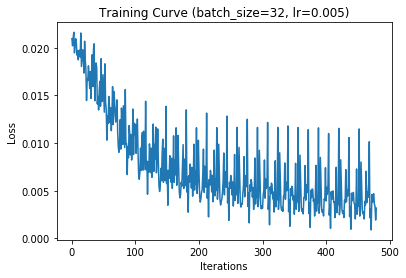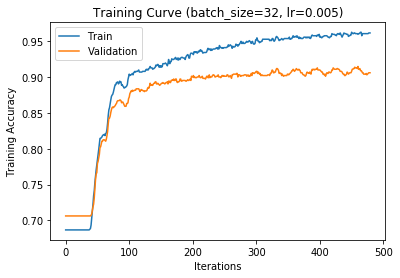Final Training Accuracy: 0.9619140625
Final Validation Accuracy: 0.90625


And the training curve for the smaller pigeon:

In :
smaller_pigeon = plot_training_curve_with_val(Pigeon(15),
batch_size=32,
lr=0.005,
num_epochs=15)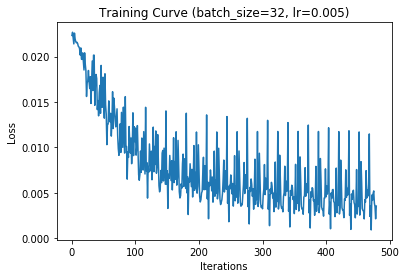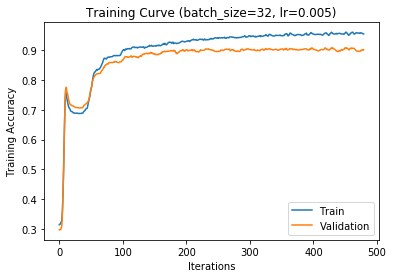Final Training Accuracy: 0.9541015625
Final Validation Accuracy: 0.9013671875


In this case, our initial choice of 30 hidden units looks quite reasonable.

## Checkpointing¶

Normally, we will train our neural network for not just one epoch, but many. Neural network training typically takes a long time, sometimes days, weeks, or even months. Our training code should therefore be robust to interruptions. That is, we should write our training code so that we can save and re-load weights.

It is good to checkpoint training progress by saving the neural network parameter values and training curve data to disk, once every few epochs. The frequency of checkpointing depends on many factors, but I recommend checkpointing every 10-30 minutes for large projects, and every few minutes for smaller ones.

Another advantage of checkpointing is that we now have one extra hyper-parameter we can tune for free: the epoch number! You may not wish to choose neural network parameter values at the end of training, and might opt to choose the parameter values at a different epoch of training.

One reason you might opt to do so is to prevent over-fitting. If your training loss is decreasing (as training progresses), but your validation loss stays the same, then your network is beginning to learn idiosyncrasies of the training set that do not generalize. Most often, we choose the earliest epoch with the lowest validation loss or error.